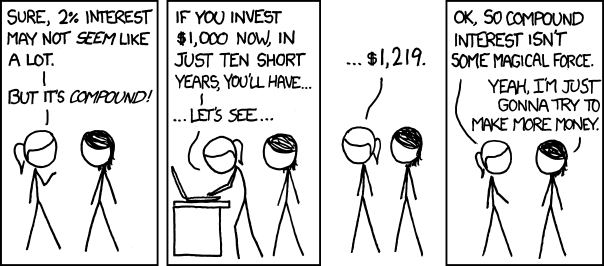# Compound Interest Calculator

Compound interest calculator, will help you quickly understand, how much you can make from your investments. If you know how much you want to invest, and what is your expected return, you can get that answer in seconds. Just provide this information and let the program calculate it for you. Explanations for the data fields are under the calculator.

 Input Data Present Value: Annual Investment: Interest Rate(%): Years of Compounding: How many times compounded annually: Results Total Invested: Total Interest: Future Value:

Definitions:

• Present Value – The amount you are starting with. You can put 0, or more here.
• Annual Investment – How much you want to add to your investment each year. It can be 0, if you want to just calculate fixed return on one off investment.
• Interest Rate(%) – How much each year you are expecting to increase your total investment.
• Years of Compounding – How many years are you planning to compound your interests.
• How many times compounded annually – Is the interest capitalised once a year, or multiple times?
• Total Interest – The amount of interest made (the money ‘earned’).
• Future Value – Total value of the investment at the end.